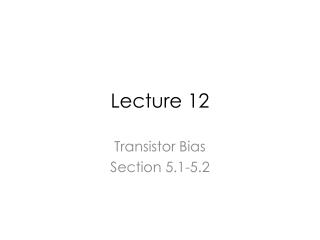# Lecture 12 - PowerPoint PPT PresentationDownload PresentationLecture 12

Lecture 12
Download Presentation## Lecture 12

- - - - - - - - - - - - - - - - - - - - - - - - - - - E N D - - - - - - - - - - - - - - - - - - - - - - - - - - -
##### Presentation Transcript

1. Lecture 12 Transistor Bias Section 5.1-5.2

2. K-30/AK-710 FM Wireless Microphone Radio Frequency Oscillator Radio Frequency Amplifier Audio Amplifier

3. Operating Point Analysis and Design

4. Simple Biasing Assumed VBE and β → IB→IC →VC Comments: IB is sensitive to VBE. IC is very sensitive to β.

5. Iteration Solution (Kick off iteration with VBE=0.7) IC=βIB

6. Example 1 Assumed: RB=1 Mohms RC=2 Kohm IS=6.734e-15 Beta=155

7. ADS Simulation IB=11.3 uA IC=1.76 mA Beta=155

8. Execute Iterations in Matlab

9. β Variation Variation of gm implies that the gain of the amplifier will vary significantly as a function of beta.

10. Resistive Divider Biasing

11. Back of the Envelope Calcuation Assume the base current is negligible, i.e. negligible compared to the current in R1. IC does not depend on Beta!

12. Negligible Base Current VBE=R3/(R1+R3)VCC VBE=5.75/(5.75+17)2.5V=0.631 V I(R1)/IB=110uA/2.10=52.38

13. Sensitivity to Component Variation 1% error in R2 leads to 14 % error in IC. 5% error in R2 leads to 85 % in IC.

14. Biasing with Emitter Degeneration If R2↑→VP↑→IE↑→VRE↑→small ∆VBE →Small ∆IC An error in VX due to inaccuracies in R1, R2 and VCC is absorbed by RE, leading to a smaller change in VBE.

15. Design Rules VRE should be > 100 mV.

16. ADS Simulation IB=12.4 uA IC=1.91 mA Beta=154

17. Sensitivity to Component Variation 1% error in R2 leads to 1.05 % error in IC. 5% error in R2 leads to 5.7 % error in IC.

18. Misc.

19. What if base current is not ignored!

20. Iterative Method Initial assumption: VBE=0.7 V